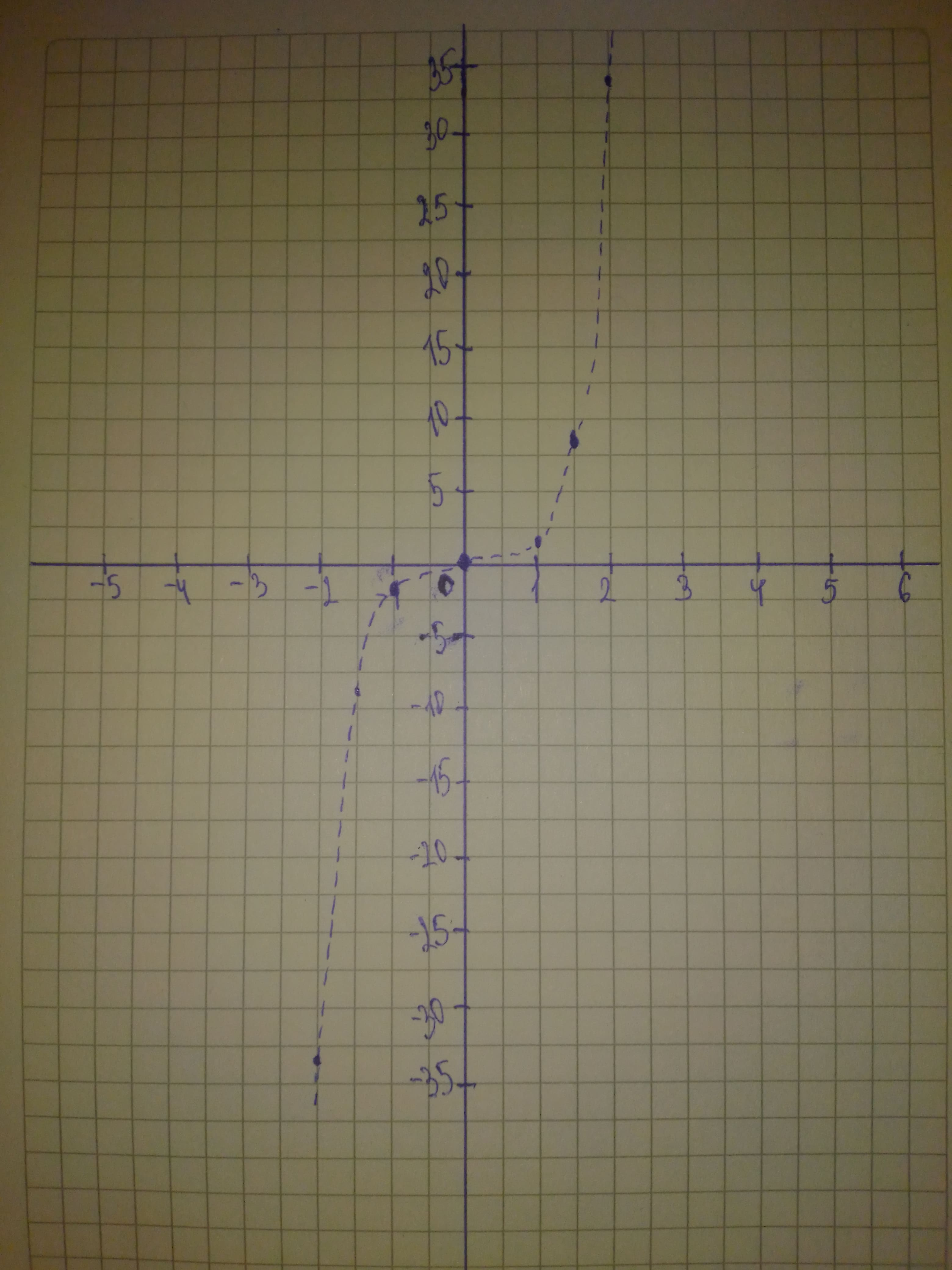# Graph the polynomial function. f(x)=x^{5}+xusagirl007A 2020-12-30 Answered
Graph the polynomial function.
$f\left(x\right)={x}^{5}+x$
You can still ask an expert for help

• Live experts 24/7
• Questions are typically answered in as fast as 30 minutes
• Personalized clear answers

Solve your problem for the price of one coffee

• Math expert for every subject
• Pay only if we can solve itLacey-May Snyder

Step 1
$f\left(x\right)={x}^{5}+x$
The leading coefficient is 1 (positive) and the degree is 5 (odd)
Therefore
and
Step 2We have step-by-step solutions for your answer!